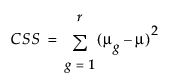Publication date: 04/12/2021

## Power

To compute power, you use the noncentral F distribution. The formula (O’Brien and Lohr 1984) is defined as follows:

Power = Prob(F > Fcrit, ν1, ν2, nc)

where:

F is distributed as the noncentral F(nc, ν1, ν2) and Fcrit = F(1-α, ν1, ν2) is the 1 - α quantile of the F distribution with ν1 and ν2 degrees of freedom.

ν1 = r -1 is the numerator df.

ν2 = r(n -1) is the denominator df.

n is the number per group.

r is the number of groups.

nc = n(CSS)/σ2 is the non-centrality parameter.is the corrected sum of squares.

μg is the mean of the gth group.

μ is the overall mean.

σ2 is estimated by the mean squared error (MSE).

Want more information? Have questions? Get answers in the JMP User Community (community.jmp.com).